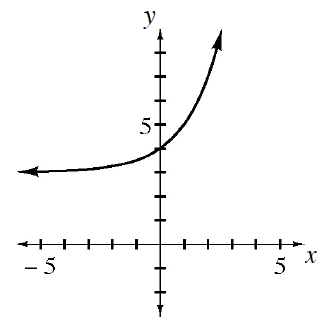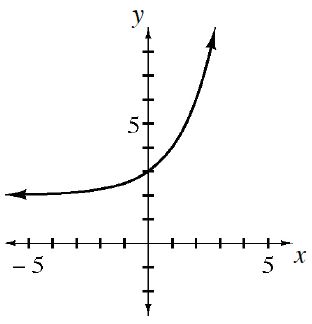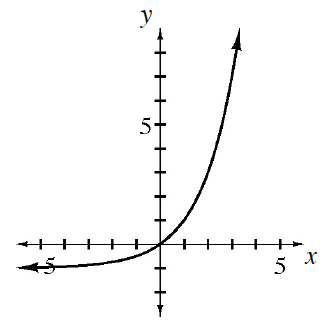### Home > INT1 > Chapter 10 > Lesson 10.2.3 > Problem10-105

10-105.

Match each equation below with its corresponding graph. Can you do this without making any tables?​

1. $y=2^x-1$

1. $y=2^x+3$

1. $y=2^x+2$

1)2)3)Consider the y-intercept for each function.

Considering equation (a). The y-intercept is calculated by letting $x = 0$.
So $y = 2^{0}−1$ so y = 0 when $x = 0$.
Which graph has $(0,0)$ on it?